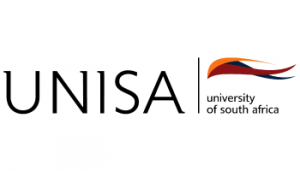relevant institution directly for course information.

# Register Here

FOR ALL THE LATEST OFFERS
AND SPECIALS NOW

Register
What are you looking for?# COURSE: BSC IN MATHEMATICAL AND STATISTICAL SC...N/A
###### Duration:
3 Years Full Time / Full Time / Part Time
This degree ensures that students have a broad theoretical and practical understanding of operations research, mathematics and applied mathematics in a decision-making environment. An added benefit is that it integrates the principles behind various mathematical techniques with their practical application in the business world.

Duration: 3 years part time

#### Compulsory Subjects

##### First year subjects:

• Introduction to the business world

• Modelling in a financial environment

• Descriptive statistics and probability

• Data analysis and inference

• Pre-calculus Mathematics

• Calculus

• Linear algebra

• Applied linear algebra

• Mathematical modelling

• Comprehension skills for science

• End-user Computing

• Introduction to programming

##### Second year subjects:

• Mathematical programming

• Rational decision making

• Financial modelling

• Distribution theory

• Linear algebra

• Introduction to discrete mathematics

• Differential equations

• Numerical methods

##### Third year subjects:

• Partial differential equations

• Numerical methods

• Ordinary differential equations

• Discrete mathematics: Combinatorics

Careers: statistical modeller, mathematical modeller.

Source: www.unisa.ac.za